Deepak Scored 45->99%ile with Bounce Back Crack Course. You can do it too!

# laws of motion class 11 questions with answers: Physics NLM Chapter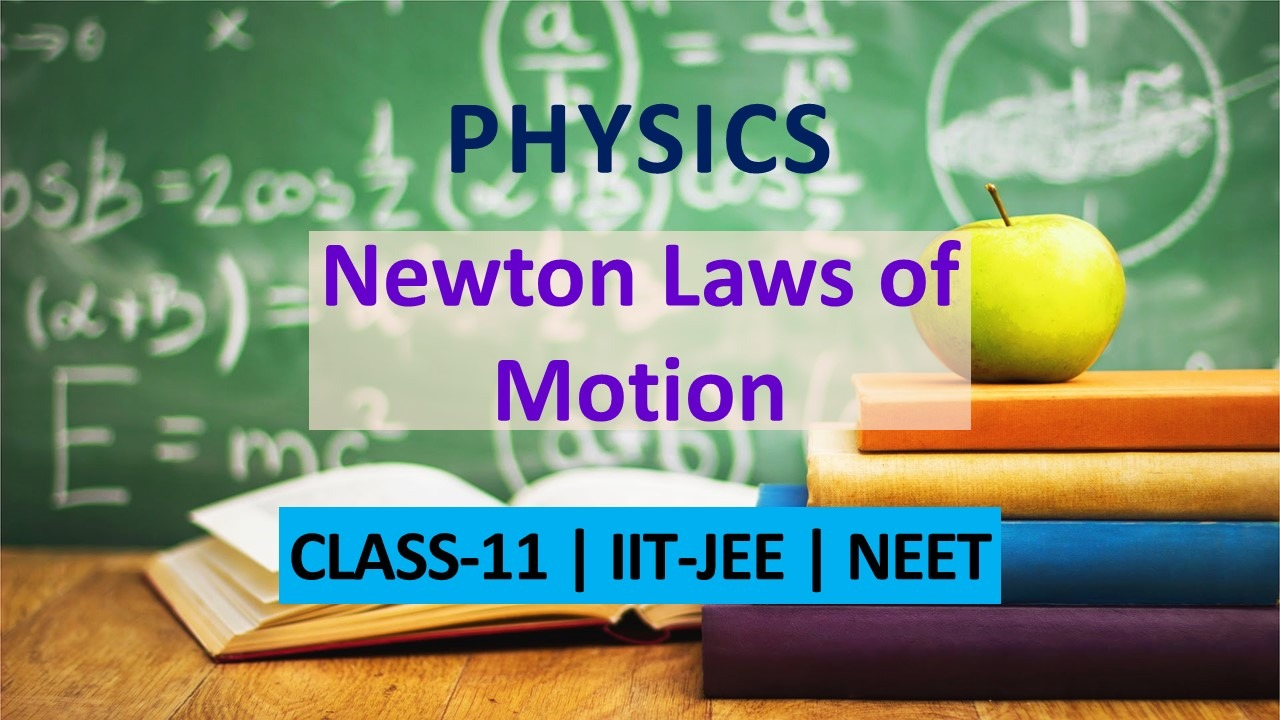thGet important laws of motion class 11 questions with answers developed by expert faculties to provide affordable and quality education to students.  View class 11 Physics important questions with answers for exam point of view from the topic Nwton's Laws of Motion fully solved. These important questions will play significant role in clearing concepts of the chapter. This question bank is designed by keeping NCERT in mind and the questions are updated with respect to upcoming Board exams. You will get here all the important questions with their answers for Class 11& 12 chapters. In this article student's will get laws of motion questions with answers which is an important topic of Class 11 Physics.
###### Click Here for Detailed Notes of Newton's Laws of Motion along with other chapters and subjects.
Q. Name one factor on which the inertia of a body depends.
Ans.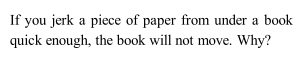Q. If you jerk a piece of paper from under a book quick enough, the book will not move. Why?
Ans.Q. Is a bus moving along a circular track, an inertial frame of reference?
Ans.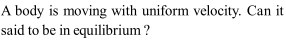Q. A body is moving with uniform velocity. Can it said to be in equilibrium?
Ans.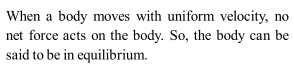Q. Why do we beat dusty blanket with a stick to remove dust particle?
Ans.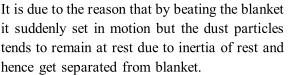Q. An astronaut accidentally gets separated out of his small spaceship accelerating in inter-stellar space at a constant rate of . What is the acceleration of the astronaut the instant after the is outside the spaceship?
Ans.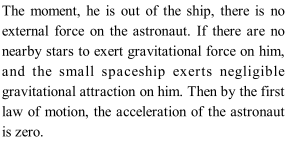Q. Why do we use shock absorbers in automobiles?
Ans.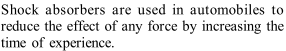Q. Why bogies of a train are provided with the buffers?
Ans.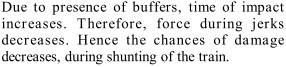Q. If a body is not at rest, the net external force acting on it cannot be zero. Is it true or false?
Ans.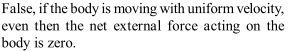Q. What is the impulsive force?
Ans.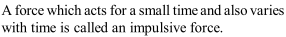Q. A thief jumps from the upper storey of a house with a load on his back. What is the force of the load on his back when the thief is in air?
Ans.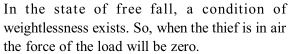Q. Why does a swimmer push the water backwards?
Ans.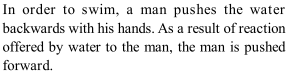Q. Can a body in linear motion be in equilibrium?
Ans.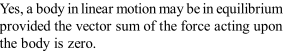Q. A uniform rope of length and mass hung from a support. What is the tension at a distance from the free end?
Ans.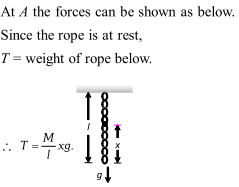Q. In the arrangement given, if the points and move down with a velocity , find the velocity of M?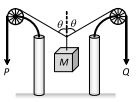Ans.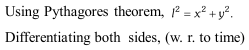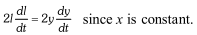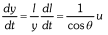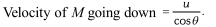Q. A soda water bottle is falling freely. Will the bubbles of gas rise in the water of the bottle?
Ans.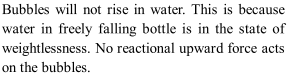Q. A bird is sitting on the floor of a wire cage and the cage is in the hands of a boy. The bird starts flying in the cage. Will the boy experience any change in the weight of the cage?
Ans.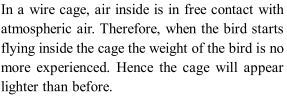Q. Air is thrown on a sail attached to a boat from an electric fan placed on the boat. Will the boat start moving?.
Ans.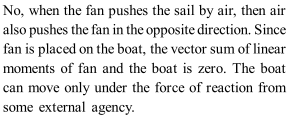Q. Is a 'single isolated force' possible in nature?
Ans.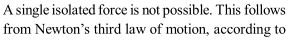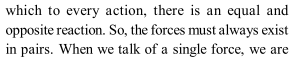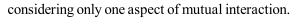Q. A disc of mass $m$ is placed on a table. A stiff spring is attached to it and is vertical. To the other end of the spring is attached a disc of negligible mass. What minimum force should be applied to the upper disc to press the spring such that the lower disc if lifted off the table when the external force is suddenly removed?
Ans.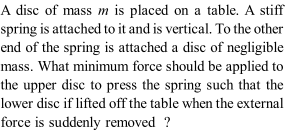Q. Explain how a man walks on the ground?
Ans.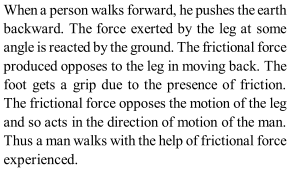Q. A man weights 70 kg. He stands on a weighting scale in a lift which is moving. (a) Upward with a uniform speed of $10 \mathrm{ms}^{-1}$. (b) Downward with a uniform acceleration of $5 \mathrm{ms}^{-2}$ with a uniform acceleration of (c) Upward with a uniform acceleration of $5 \mathrm{ms}^{-2}$. What would be the reading on the scale in each case? What would be the reading if the lift mechanism failed, and it hurtled down freely under gravity? $\left[g=10 \mathrm{ms}^{-2}\right]$
Ans.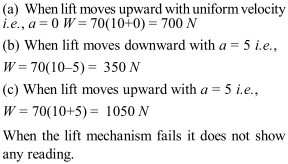Q. It is easy to catch a table tennis ball than a cricket ball, even when both are moving with the same velocity. Why?
Ans.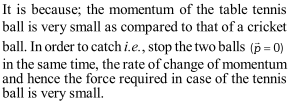Q. In which case will a rope have the greater tension (a) Two men pull the ends of the rope with forces $F$ equal in magnitude but opposite in direction. (b) One end of the rope is fastened to a fixed support and the other is pulled by a man with a force $2 F .$
Ans.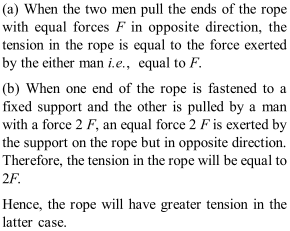Q. Ten one-rupee coins are put on the top of eachother on a table. Each coin has a mass $m$ kg. Give the magnitude and direction of (a) The force on the $7^{\text {th }} \operatorname{coin}$ (counted from the bottom) due to all the coins on its top (b) The force on the $7^{\text {th }}$ coin by the eighth coin (c) The reaction of the $6^{\text {th }}$ coin on the $7^{\text {th }}$ coin [NCERT]
Ans.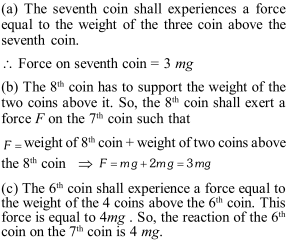Q. A force acting on a material particle of mass $m$ first grows to a maximum value $F_{m}$ and then decreases to zero. The force varies with time according to a linear law, and total time of motion is $t_{m}$. What will be the velocity of the particle at the end of this interval if the initial velocity is zero
Ans.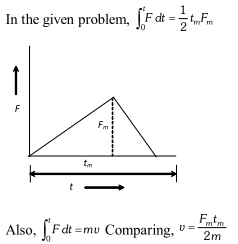Q. The assertion made by the Newton's first law ofmotion that every body continues in its state of uniform motion in the absence of external force appears to be contradicted in everyday experience. Why?
Ans.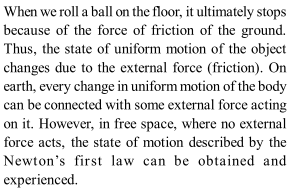Q. A stone when thrown on a glass window smashes the window pane to piece, but a bullet from the gun passes through making a clean hole. Why?
Ans.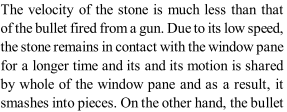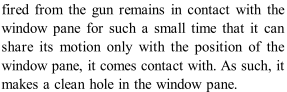Q. A block is supported by a cord $C$ from a rigid support, and another cord $D$ is attached to the bottom of the block. If you give a sudden jerk to $D,$ it will break. But if you pull on $D$ steadily, $C$ will break. Why?
Ans.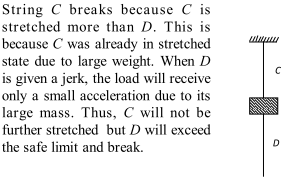Q. Why do you fall forward when a moving train decelerates to a stop and fall backward when a train accelerates from rest? What would happen if the train rounded a curve at constant speed? [NCERT]
Ans.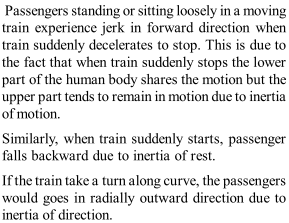Q. "It is reasonable to consider the Earth as an inertial frame for many laboratory scale terrestrial experiments, but the Earth is a non-inertial frame of reference for astronomical observation." Explain this statement. Do you see how the same frame of reference is approximately inertial for one purpose, and non-inertial for the other? [NCERT]
Ans.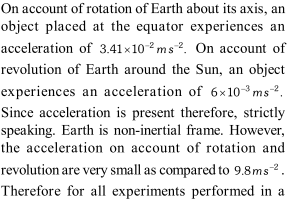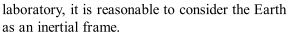Q. A mass of $6 \mathrm{kg}$ is suspended by a rope of length $2 \mathrm{m}$ from a ceiling. A force of $50 \mathrm{N}$ in the horizontal direction is applied at the mid-point of the rope, as shown in the figure. What is the angle that rope makes with the vertical in equilibrium? Neglect the mass of the rope. Given : $\mathrm{g}=10 \mathrm{ms}^{-2}$. [NCERT]
Ans.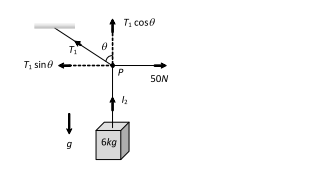Q. A piece of uniform string hangs vertically, so that its free end just touches the horizontal surface of a table. If the upper end is released, show that at any instant during fall of the string, the total force on the surface is three times the weight of that part of the string which is lying on the surface. [NCERT]
Ans.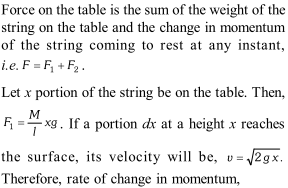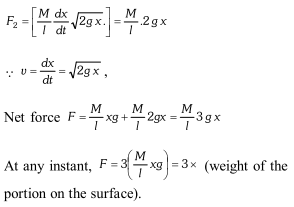Q. With what acceleration ' $a^{\prime}$ should a box descend so that a block of mass $M$ placed in it exerts a force $\frac{\mathrm{Mg}}{4}$ on the floor of the box?
Ans.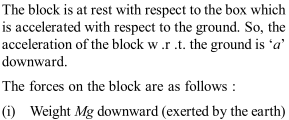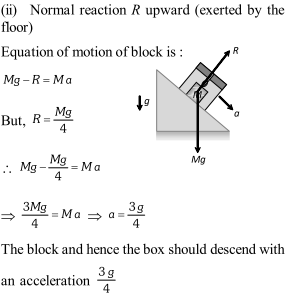Q. The pulley arrangements of figures $(a)$ and $(b)$ are identical. The mass of the rope is negligible. In figure $(a),$ the mass $m$ is lifted up by attactating a mass $2 m$ to the other end of the rope. In Figure (b), $m$ is lifted up by pulling the other end of the rope with a constant downward force $F=2 m g$. Calculate the accelerations in the two cases.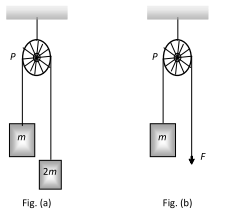Ans.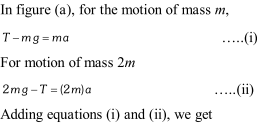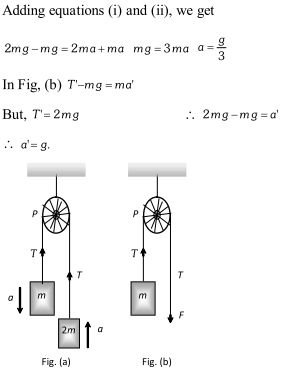Q. A monkey of mass 40 kg climbs on a rope which can withstand a maximum tension of $600 N .$ In which of the following cases will the rope break: the monkey (a) Climbs up with an acceleration of $6 \mathrm{ms}^{-2}$ (b) Climbs down with an acceleration of $4 \mathrm{ms}^{-2}$ (c) Climbs up with a uniform speed of $5 \mathrm{ms}^{-1}$ (d) Falls down the rope nearly freely under gravity Take $g=10 \mathrm{ms}^{-2}$. Ignore the mass of the rope. [NCERT] (new)
Ans.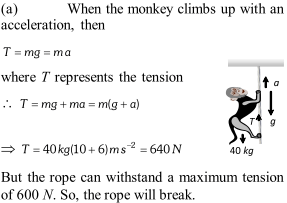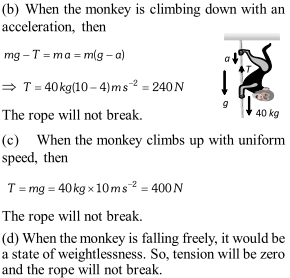Q. Give the magnitude and direction of the net force acting on (a) A drop of rain falling down with a constant speed (b) A cork of mass 10 g floating on water (c) A kite skilfully held stationary in the sky (d) A car moving with a constant velocity of $30 \mathrm{km}$ (e) A high-speed electron in space far from all gravitating objects and free of electric and magnetic fields.
Ans.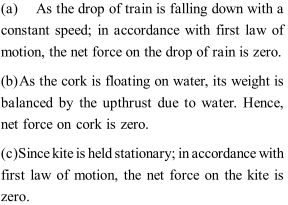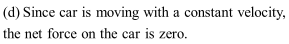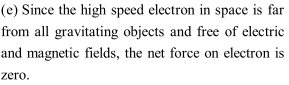Q. For ordinary terrestrial experiments, which of the observers below are inertial and which non-inertial? (a) A child revolving in a "giant wheel" (b) A driver in a sport car moving with a constant high speed of $200 \mathrm{km} \mathrm{h}^{-1}$ on a straight road, (c) The pilot of an aeroplane which is taking off, (d) A cyclist negotiating a sharp turn, (e) The guard of a train which is slowing down to stop at a station? [NCERT]
Ans.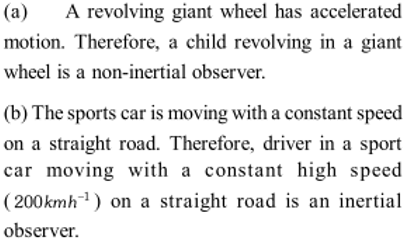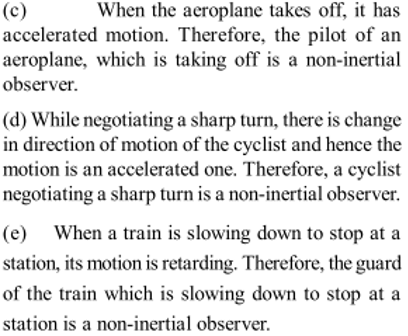Q. Explain, why (a) A horse cannot pull a cart and run in empty space. (b)Is it easier to pull a lawn mower than to push it (c) Does a cricketer move his hands backward when holding a catch.
Ans.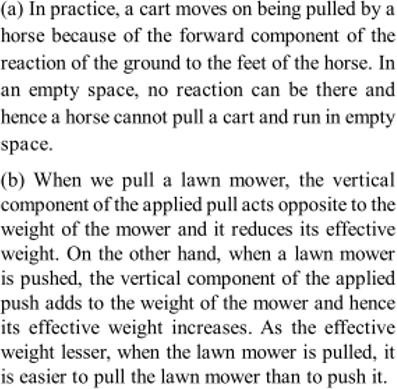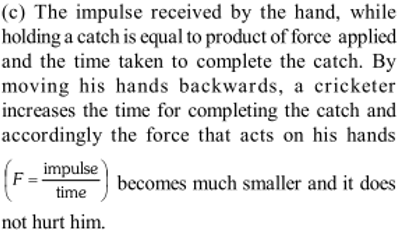Q. Fig. Shows the position-time graph of a particle of mass 0.04 kg. Suggest a suitable physical context for this motion. What is the time between two consecutive impulses received by the particle $?$ What is the magnitude of each impulse?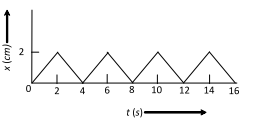[NCERT]
Ans.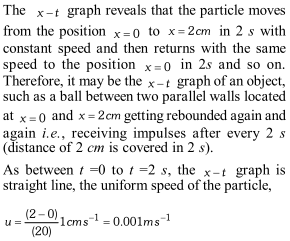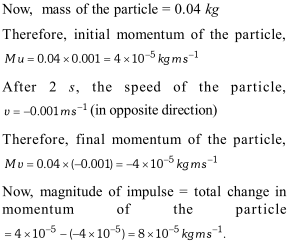Q. Two identical billiard balls strike a rigid wall with the same speed but at different angles, and get reflected without any loss of speed, as shown in the figure below. What is (i) the direction of the force on the wall due to each ball? and (ii) the ratio of the magnitudes of impulses imparted on the two balls by the wall? [NCERT]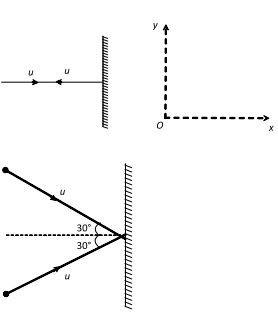Ans.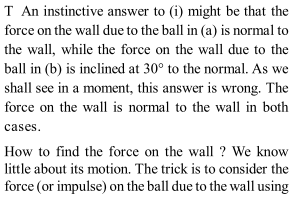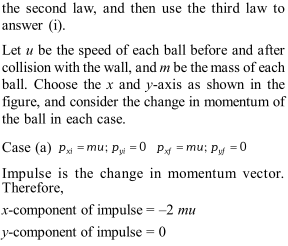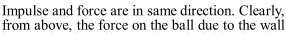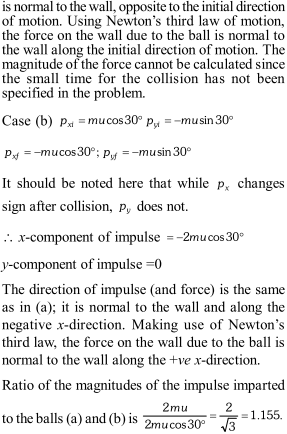Q. A light string carrying a small bob hangs from the roof of a vehicle as shown in the figure. If the vehicle moves in a horizontal straight line with a constant acceleration of $2.4 \mathrm{ms}^{-2}$ from left to right, determine the angle, which the string makes with the vertical.
Ans.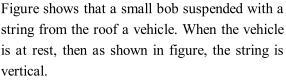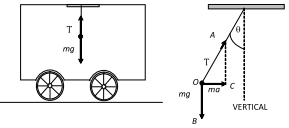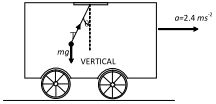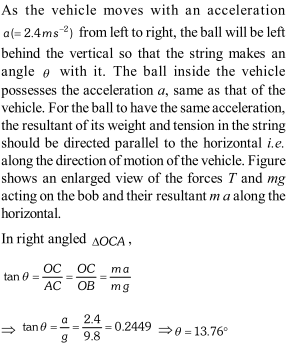Q. Two masses 7 kg and 12 kg are connected at the two ends of a light inextensible strings that passes over frictionless pulley. Find the acceleration of the masses and the tension in the string, when the masses are released.
Ans.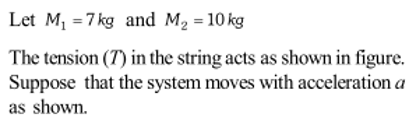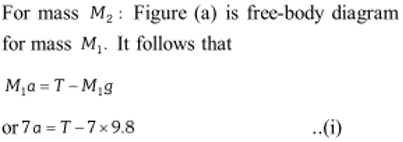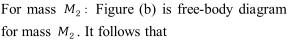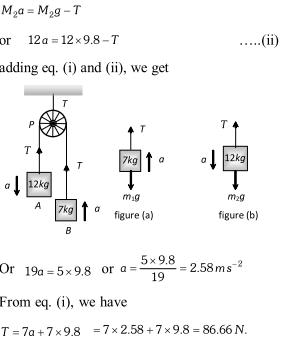Q. Two pulley of masses $12 \mathrm{kg}$ and $8 \mathrm{kg}$ are $\mathrm{d} \mathrm{e}$ connected by a fine string hanging over a fixed pulley as shown. Over the latter is hung a fined string with masses $4 \mathrm{kg}$ and $M .$ Over the $12 \mathrm{kg}$ pulley is hung another fine string with masses 3 $\mathrm{kg}$ and $6 \mathrm{kg}$. Calculate $M$ so that the string overthe fixed pulley remains stationary.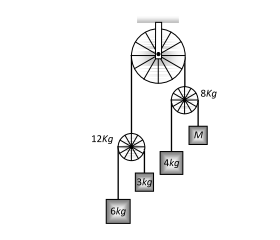Ans.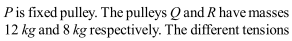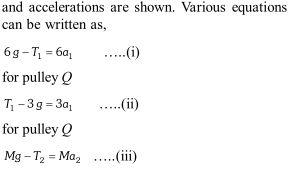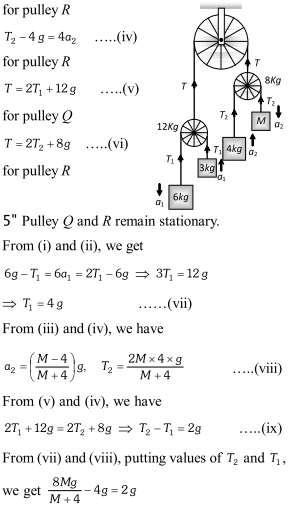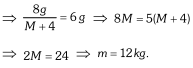Q. One end of a string is attached to a 6 kg mass on a smooth horizontal table. The string passes, the edge of the table, and to its other end is attached a light smooth pulley. Over this pulley passes another string to the ends of which are attached masses of $4 \mathrm{kg}$ and $2 \mathrm{kg}$ respectively as shown. Show that the $6 \mathrm{kg}$ mass moves with an acceleration of $\frac{8 \mathrm{g}}{17}$.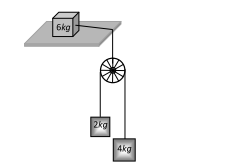Ans.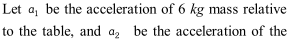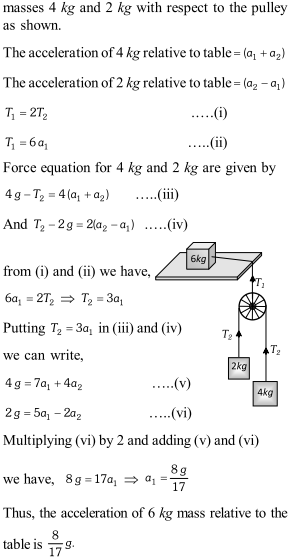Q. A wooden block of mass 2 kg rests on a soft horizontal floor. When an iron cylinder of mass $25 \mathrm{kg}$ is placed on top of the block, the floor yields steadily and the block and the cylinder together go down with an acceleration of $0.1 \mathrm{ms}^{-2}$. What is the action of the block on the floor (a) before and (b) after the floor yields? Take $\mathrm{g}=10 \mathrm{ms}^{-2}$. [NCERT]
Ans.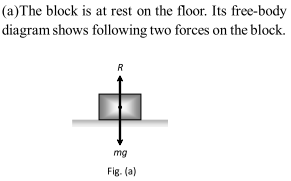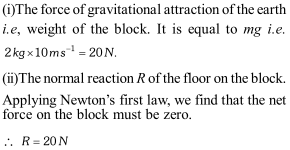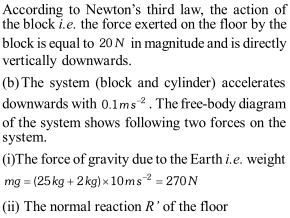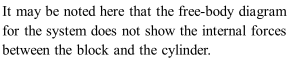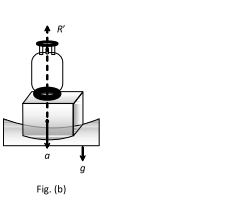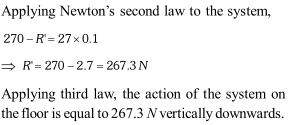eSaral provides you complete edge to prepare for Board and Competitive Exams like JEE, NEET, BITSAT, etc. We have transformed classroom in such a way that a student can study anytime anywhere. With the help of AI we have made the learning Personalized, adaptive and accessible for each and every one. You will get other chapters' and subjects' important questions with answers for Class 11 & 12 science stream. Visit eSaral Website to download or view free study material for JEE & NEET. Also get to know about the strategies to Crack Exam in limited time period.

#### Manikanta - Nov. 10, 2020, 9:53 p.m.

Its great to learn here. But in my opinion more than the theory part the problems part must be more and the theory part is also fantastic i want just one sum but here i learned full in theory even some of them i didnt knew. If there are more number of problems it may be one off the best learning logestics. Thank You

#### SouLReGaLToS - Sept. 16, 2020, 5:36 p.m.

THIS IS DAMM GOOD TO PRACTICE FOR NEWTON'S LAWS OF MOTION AS IT HAS ALL THE TYPES OF QUESTION

#### charmi - Sept. 7, 2020, 4:28 p.m.

It's good

None
Free Study Material